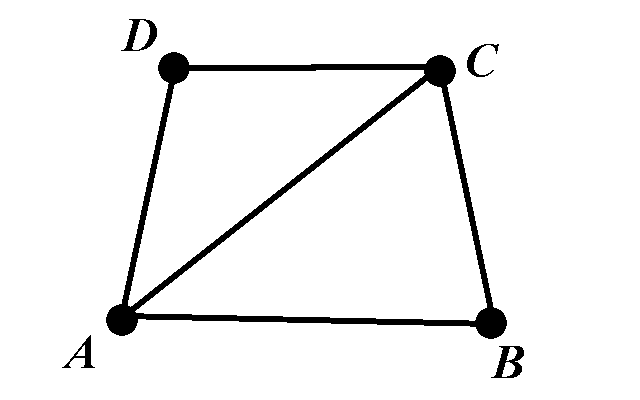MTH4140 Home

# Chromatic number

## Proper colorings and chromatic numbers

Definition

The chromatic number $$\chi(G)$$ of the graph $$G=(V,E)$$ is the smallest integer $$k$$ for which the vertices from $$V$$ can be colored with $$k$$ colors such that no two neighbors share the same color.

Let us calculate the chromatic number of the graph from the picture below:We can color the graph with $$3$$ colors. The vertices $$D$$ and $$B$$ can have the same color. Let us call that color $$1$$. This means that the vertices $$B$$ and $$D$$ have the color $$1$$. The vertex $$A$$ can be of color $$2$$ and the vertex $$C$$ is of color $$3$$. Therefore, the graph can be colored with $$3$$ colors.

We now need to prove that $$3$$ is the smallest number of colors. In other words, we must prove that it is not possible to color the vertices of the graph with $$2$$ colors. To see this, observe that the vertices $$A$$, $$B$$, and $$C$$ form a complete subgraph: every one of $$A$$, $$B$$, and $$C$$ is connected to every other. This means that they all must have different colors, which implies that $$2$$ colors cannot be used to paint these three vertices.

We will now provide a formal definition of coloring.

Definition

A proper coloring of the graph $$G=(V,E)$$ with $$k$$ colors is any function $$f:V\to \{1,2,\dots, k\}$$ that satisfies:

For every two vertices $$u,v\in V$$ such that $$(u,v)\in E$$, the values $$f(u)$$ and $$f(e)$$ are different.

Then the chromatic number $$\chi(G)$$ can be defined as $\chi(G)=\min\left\{k:\mbox{There exists a proper coloring of }G \mbox{ with }k\mbox{ colors.}\right\}.$

## Parameters of the graph

Definition

Let $$G=(V,E)$$ be a graph.

• $$\Delta(G)$$ is the maximum of degrees of the vertices. $\Delta(G)=\max\left\{d(v):v\in V\right\}.$

• $$\delta(G)$$ is the minimum of degrees of the vertices. $\delta(G)=\min\left\{d(v):v\in V\right\}.$

• $$\alpha(G)$$ is the independence number - the maximum of the size of an independent set. A set of vertices $$I\subseteq V$$ is called independent if no two vertices from $$I$$ are adjacent. $\alpha(G)=\max\left\{|I|:I\subseteq V, \; I \mbox{ is an independent set}\right\}.$

• $$\omega(G)$$ is the clique number - the maximum size of a complete subgraph. $\omega(G)=\max\left\{m:\mbox{ the complete graph }K_m\mbox{ is a subgraph of }G\right\}.$

Exercise

Calculate $$\delta(G)$$, $$\Delta(G)$$, $$\alpha(G)$$ and $$\omega(G)$$ for the graph from the picture above.

## Bounds on the chromatic number

Theorem 1

The inequality $$\chi(G)\leq 1+\Delta(G)$$ holds for every simple graph $$G$$.

Theorem 2

Assume that the chromatic number of the simple graph $$H$$ is equal to $$k$$ and that $$H$$ is a $$k$$-critical graph. Then the following inequality holds $\delta(H)\geq k-1.$

Theorem 3

The following inequality holds for every simple graph $$G$$: $\chi(G)\leq 1+ \max\left\{\delta(H): H\mbox{ is a subgraph of } G\right\}.$

Theorem 4

Assume that the simple graph $$G$$ has $$n$$ vertices and that their degrees are $d_1\geq d_2\geq\cdots \geq d_n$ in non-decreasing order. The following inequality holds $\chi(G)\leq 1+\max_i\min\left\{i-1,d_i\right\}.$

Theorem 5

Assume that $$G$$ is a simple graph. Then the following inequality holds $$\chi(G)\geq \frac{n(G)}{\alpha(G)}$$.